Representation of Rational Numbers on the Number Line

# Representation of Rational Numbers on the Number Line Notes | Study Mathematics (Maths) Class 8 - Class 8

## Document Description: Representation of Rational Numbers on the Number Line for Class 8 2022 is part of Mathematics (Maths) Class 8 preparation. The notes and questions for Representation of Rational Numbers on the Number Line have been prepared according to the Class 8 exam syllabus. Information about Representation of Rational Numbers on the Number Line covers topics like and Representation of Rational Numbers on the Number Line Example, for Class 8 2022 Exam. Find important definitions, questions, notes, meanings, examples, exercises and tests below for Representation of Rational Numbers on the Number Line.

Introduction of Representation of Rational Numbers on the Number Line in English is available as part of our Mathematics (Maths) Class 8 for Class 8 & Representation of Rational Numbers on the Number Line in Hindi for Mathematics (Maths) Class 8 course. Download more important topics related with notes, lectures and mock test series for Class 8 Exam by signing up for free. Class 8: Representation of Rational Numbers on the Number Line Notes | Study Mathematics (Maths) Class 8 - Class 8
 1 Crore+ students have signed up on EduRev. Have you?

A rational number is any real number that can be expressed as a simple fraction or ratio. By definition, a rational number is a number which can be written in the form of p/q, where p and q are any two integers and q not equal to zero (q ≠ 0). Rational numbers can be positive or negative. If we take a closer look at counting numbers, all of them are rational numbers with denominator 1. The set of rational numbers is denoted by the letter “Q”.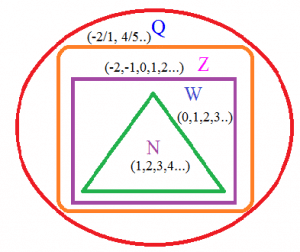Real numbers

Representation of Rational Numbers on a Number Line
Like the representation of the whole numbers, integers on the number line, the rational numbers can also be represented on the number line. In the number line, the number zero (0) is called the origin. All the positive values are represented on the right side of the origin, and the negative values are represented on the left side of the origin. Now, let us learn how to represent the rational numbers on the number line.
The representation of rational numbers can be classified into two different types. The rational number may be in proper fraction or improper fraction.
If it is a proper fraction, the numerator value is less than the denominator value, and hence the given rational number should be less than 1 and greater than zero, and we can easily represent the given rational number on the number line.
If it is an improper fraction, the numerator value is greater than the denominator value, and hence the given rational number should be greater than 1. So, in this case, first, convert the given improper fraction into a mixed fraction. This conversion helps to locate the exact position of the given fraction on the number line. It helps to know between which integer does the fraction lie.
Procedure to Represent the Rational Number on the Number Line:

• Draw a line and locate the point ‘0’. This point is known as the origin.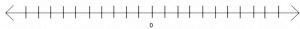• If the given number is positive, mark it on the right side of the origin. If it is a negative number, mark it on the left side of zero.
• Divide each unit into the values which are equal to the denominator of the fraction. For example: representing 4/5 on the number line, you need to divide each unit into 5 subunits.

Example: Represent 2/3 on a number line.
Solution: 2/3 is a positive rational number, and it is known that 2/3 is less than 1 and greater than 0. Therefore, 2/3 lies between 0 and 1 on the number line.
Here, the denominator is 3 so we will divide each unit length into 3 subunits between 0 and 1.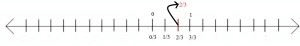Rational Number Representation Using Successive Magnification
We can represent this decimal expansion on the number line through the process of successive magnification.
We know every rational number can be expressed as decimal expansions. Here,
⅔= 0.6666666666666667
Step 1: Locate 0.6 on the number line. 0.6 lies between 0 and 1 on the number line.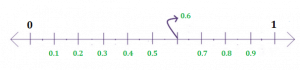Step 2: Now, represent 0.66 on the number line. As we know 0.66 lies between 0.60 and 0.70 on the number line.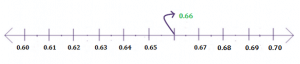Step 3: Finally, represent 0.666 on the number line. The value of 0.666 lies between 0.66 and 0.67.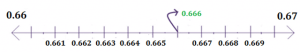By magnifying the numbers between two other numbers on the number line, we can represent the decimal expansion of rational numbers easily.

The document Representation of Rational Numbers on the Number Line Notes | Study Mathematics (Maths) Class 8 - Class 8 is a part of the Class 8 Course Mathematics (Maths) Class 8.
All you need of Class 8 at this link: Class 8

## Mathematics (Maths) Class 8

187 videos|323 docs|48 tests
 Use Code STAYHOME200 and get INR 200 additional OFF

## Mathematics (Maths) Class 8

187 videos|323 docs|48 tests

Track your progress, build streaks, highlight & save important lessons and more!

,

,

,

,

,

,

,

,

,

,

,

,

,

,

,

,

,

,

,

,

,

;